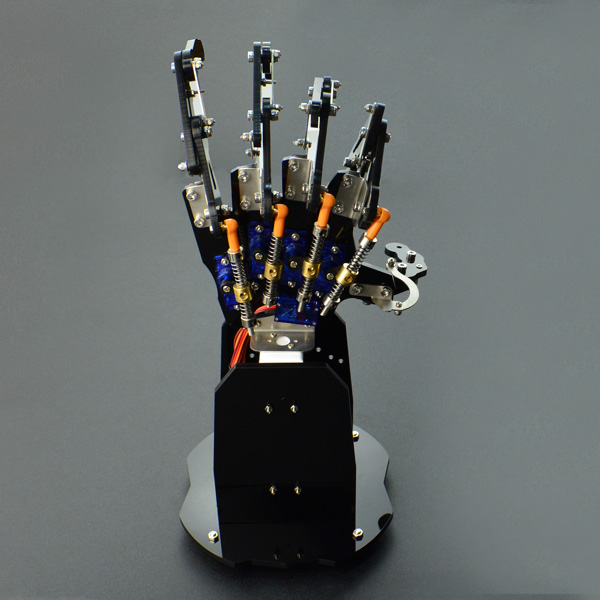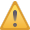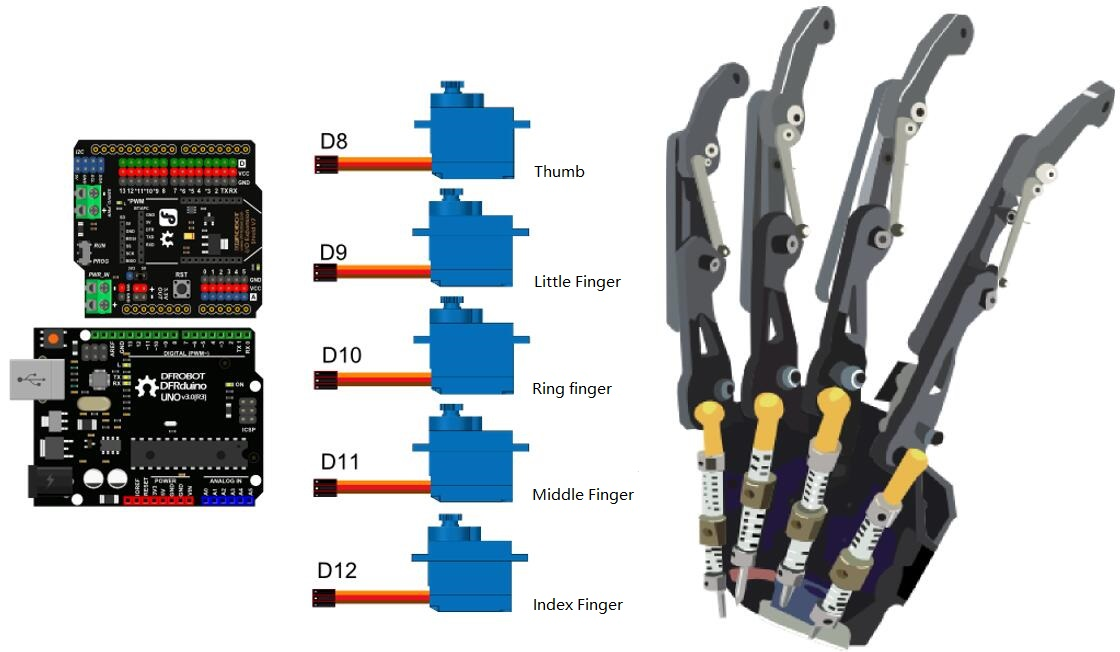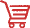Introduction

The bionic robot hand is made up of acrylic material. It consists of 5 micro metal servos, joints and one hand base. The special mechanical structure makes every finger can be controlled separately, all can move in certain range. And every finger has spring damping structure, it will protect the robotic hand from the mechanical stress effectively. With 24 channel Veyron servo driver, all actions can be controlled via PC software, supporting online debug and wireless control. The bionic robot hand can be controlled by Arduino and other Servo controllers. It can grab 500g object, and it is the best option for DIY robot hand demonstration. The servo can be connected directly to the arduino IO expansion shield or the Romeo robot microcontroller, and arduino servo library makes it easier to use.Note: Please be careful with the servo control, even if there is damping structures on each finger, the non-standard operation will still damage the servos.

Specification

• Operating Voltage: 4.8-6V
• Control Signal: 1.0ms2.0ms (45135°)
• Operating Current: 2A
• Servo Torque: 2.4kg*cm (4.8v) 2.8kg*cm (6v)
• Servo Speed: 0.11 "/ 60 ° (4.8v) 0.09" / 60 ° (6.0 V)
• Operating Temperature: 0 ~ 55 ℃
• Cable: Brown <-> GND
• Red <-> VCC
• Orange<->Signal
• Servo Dimension: 23 * 12.2 * 29mm /0.91*0.48*1.14 inches
• Hand Dimension: 330 mm / 12.99 inches (Height)
• Servo net weight: 14g
• Weight: 896g (Whole kit)

Tutorial

In this tutorial, we'll demonstrate a simple way to control the robot hand.

Connection DiagramSample Code

``````/*!
@file ROB0142.ino
@brief Bionic Robot Hand
@n [Get the module here]()
@n This example show 0-9 the 10 Numbers.
@n [Connection and Diagram]()

@author: (ju.li@dfrobot.com)
@version  V1.0
@date  2017-03-01
*/

#include <Servo.h>

Servo Saservo;
Servo Sbservo;
Servo Scservo;
Servo Sdservo;
Servo Seservo;

int Sa = 90;  // default Position
int Sb = 50;  //
int Sc = 40;  //
int Sd = 50;  //
int Se = 50;  //

void setup()
{
Serial.begin(9600);  //Serial Baudrate
Saservo.attach(8);   //thumb servo
Sbservo.attach(9);   //little finger servo
Scservo.attach(10);  //ring finger servo
Sdservo.attach(11);  //middle finger servo
Seservo.attach(12);  //index finger servo

Saservo.write(90);   //thumb
Sbservo.write(50);   //little finger
Scservo.write(40);   //ring finger
Sdservo.write(50);   //middle finger
Seservo.write(50);   //index finger
delay(1000);
}

void loop()
{
char cc;  //
while (Serial.available() > 0)  //
{

if (cc == 'a')  //
{
for (Sa = 90; Sa <= 160; Sa += 1) //thumb
{
Saservo.write(Sa);
delay(15);
}

for (Sb = 50; Sb <= 150; Sb += 1) //little finger
{
Sbservo.write(Sb);
delay(15);
}

for (Sc = 40; Sc <= 130; Sc += 1) //ring finger
{
Scservo.write(Sc);
delay(15);
}

for (Sd = 50; Sd <= 150; Sd += 1) //middle finger
{
Sdservo.write(Sd);
delay(15);
}

for (Se = 50; Se <= 140; Se += 1) //index finger
{
Seservo.write(Se);
delay(15);
}
delay(1000);

//close the fingers
for (Sa = 160; Sa >= 90; Sa -= 1)
{
Saservo.write(Sa);
delay(15);
}

for (Sb = 150; Sb >= 50; Sb -= 1)
{
Sbservo.write(Sb);
delay(15);
}

for (Sc = 130; Sc >= 40; Sc -= 1)
{
Scservo.write(Sc);
delay(15);
}

for (Sd = 150; Sd >= 50; Sd -= 1)
{
Sdservo.write(Sd);
delay(15);
}

for (Se = 140; Se >= 50; Se -= 1)
{
Seservo.write(Se);
delay(15);
}

}
//9
if (cc == '9')
{
Saservo.write(90);//thumb
Sbservo.write(50);//little finger
Scservo.write(40);//ring finger
Sdservo.write(50);//middle finger
Seservo.write(110);//index finger
}

//8
if (cc == '8')
{
Saservo.write(160);//thumb
Sbservo.write(50);//little finger
Scservo.write(40);//ring finger
Sdservo.write(50);//middle finger
Seservo.write(140);//index finger
}

//7
if (cc == '7')
{
Saservo.write(160);//thumb
Sbservo.write(50);//little finger
Scservo.write(40);//ring finger
Sdservo.write(150);//middle finger
Seservo.write(140);//index finger
}

//6
if (cc == '6')
{
Saservo.write(160);//thumb
Sbservo.write(150);//little finger
Scservo.write(40);//ring finger
Sdservo.write(50);//middle finger
Seservo.write(50);//index finger
}

//5
if (cc == '5')
{
Saservo.write(160);//thumb
Sbservo.write(150);//little finger
Scservo.write(130);//ring finger
Sdservo.write(150);//middle finger
Seservo.write(140);//index finger
}

//4
if (cc == '4')
{
Saservo.write(90);//thumb
Sbservo.write(150);//little finger
Scservo.write(130);//ring finger
Sdservo.write(150);//middle finger
Seservo.write(140);//index finger
}

//3
if (cc == '3')
{
Saservo.write(90);//thumb
Sbservo.write(50);//little finger
Scservo.write(130);//ring finger
Sdservo.write(150);//middle finger
Seservo.write(140);//index finger
}

//2
if (cc == '2')
{
Saservo.write(90);//thumb
Sbservo.write(50);//little finger
Scservo.write(40);//ring finger
Sdservo.write(150);//middle finger
Seservo.write(140);//index finger
}

//1
if (cc == '1')
{
Saservo.write(90);//thumb
Sbservo.write(50);//little finger
Scservo.write(40);//ring finger
Sdservo.write(50);//middle finger
Seservo.write(140);//index finger
}

//0
if (cc == '0')
{
Saservo.write(90);//thumb
Sbservo.write(50);//little finger
Scservo.write(40);//ring finger
Sdservo.write(50);//middle finger
Seservo.write(50);//index finger
}

}
}``````

Expected Results

• a: all fingers open; all fingers closed
• 0: show gesture "0"
• 1: show gesture "1"
• 2: show gesture "2"
• 3: show gesture "3"
• 4: show gesture "4"
• 5: show gesture "5"
• 6: show gesture "6"
• 7: show gesture "7"
• 8: show gesture "8"
• 9: show gesture "9"

FAQ

For any questions, advice or cool ideas to share, please visit the DFRobot Forum.Get [link Bionic Robot Hand (Left)] & [link Bionic Robot Hand (Right)] from DFRobot Store or DFRobot Distributor.